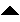Next: Convolutions and Digital Filters Up: number8 Previous: Introduction

# The Z Transform

We will start by defining a transform function that at first looks so trivial that it appears that it cannot possibly be useful. Let us assume that data is digitized at a uniform sampling rate. Say our data consisted of the sequence of points: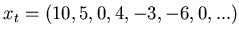(1)

We can represent this data as a polynomial: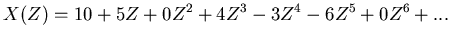(2)

This polynomial is called the Z transform of the time series. The trick with Z transforms is how we interpret what the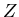actually represents. Theis called the unit delay operator because of the following, if we take (2) and multiply it bywe get,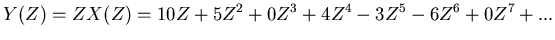(3)

which becomes the untransformed series: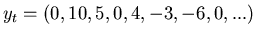which is just the original time series delayed by one unit of time (Be careful if you try to look the Z transform up in a book. In a variation of the pi throwing contest that I mentioned a few columns back, different authors use different sign conventions on the Z transform polynomial.).

This innocent looking transform and delay operator turn out to be very powerful. Consider the following, suppose that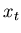represents the response of the ground to an explosion (which we will call the impulse response). If another explosion occurred 10 time units later, then the signal that we would see is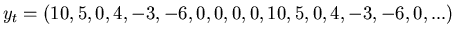which can be represented by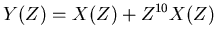If the second explosion was half the strength of the first, then we'd write,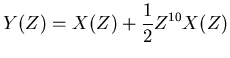If the second was a half strength implosion, then the sign is just reversed,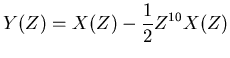If the second signal came in overlapping the first (say at time 4) then the numbers in the series is a bit messy looking,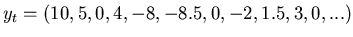but rest assured this series can be written out as having the transform,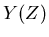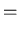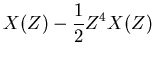(4)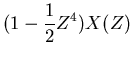(5)

A Forth function to do this kind of factorization is shown in listing 1. This code has been contributed to the Forth Scientific Library and is awaiting review for its acceptance. (Volunteers for doing code reviews are eagerly accepted!).

In general we can do this for an arbitrarily complicated sequence of explosions and implosions as long as the response of the earth remains linear (an example of a nonlinear response would be the state of the ground within a mile or so of a nuclear explosion). So suppose we have a sequence consisting of an explosion at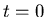, a half strength implosion at time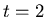and a quarter strength explosion at time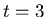,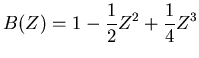(6)

then,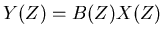(7)

Now without doing any integrals, and just manipulating polynomials we have done the convolution theorem,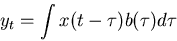(8)

Both equations (7) and (8) state that the signal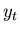is the convolution ofand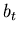, but the Z transform version just involves simple manipulation of polynomials. Listing 2 provides an implementation of convolution algorithms, this code is also an unreviewed contribution to the Forth Scientific Library.Next: Convolutions and Digital Filters Up: number8 Previous: Introduction
Skip Carter 2008-08-20